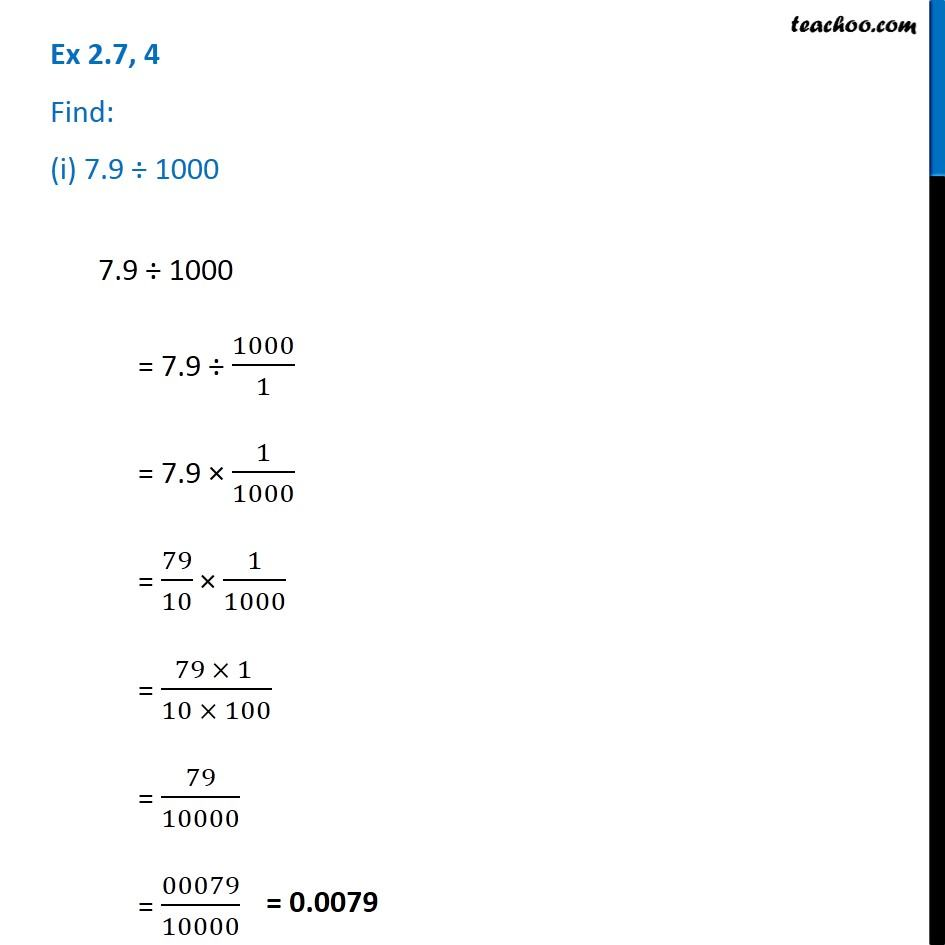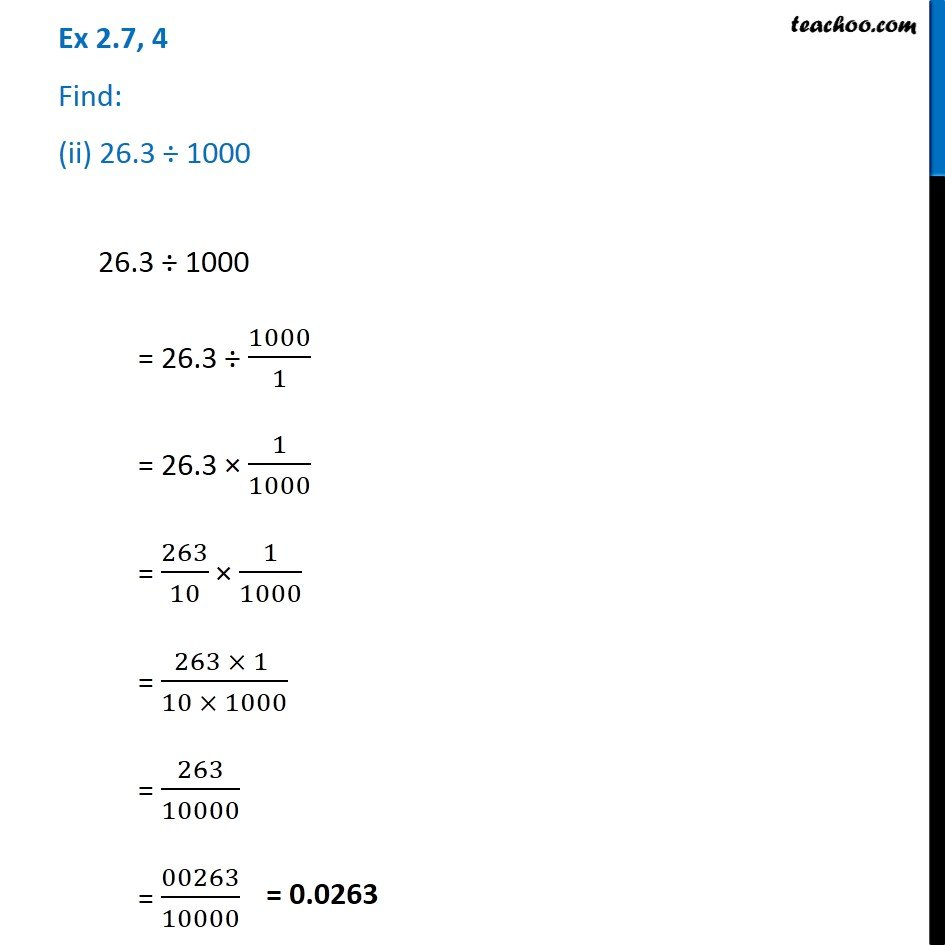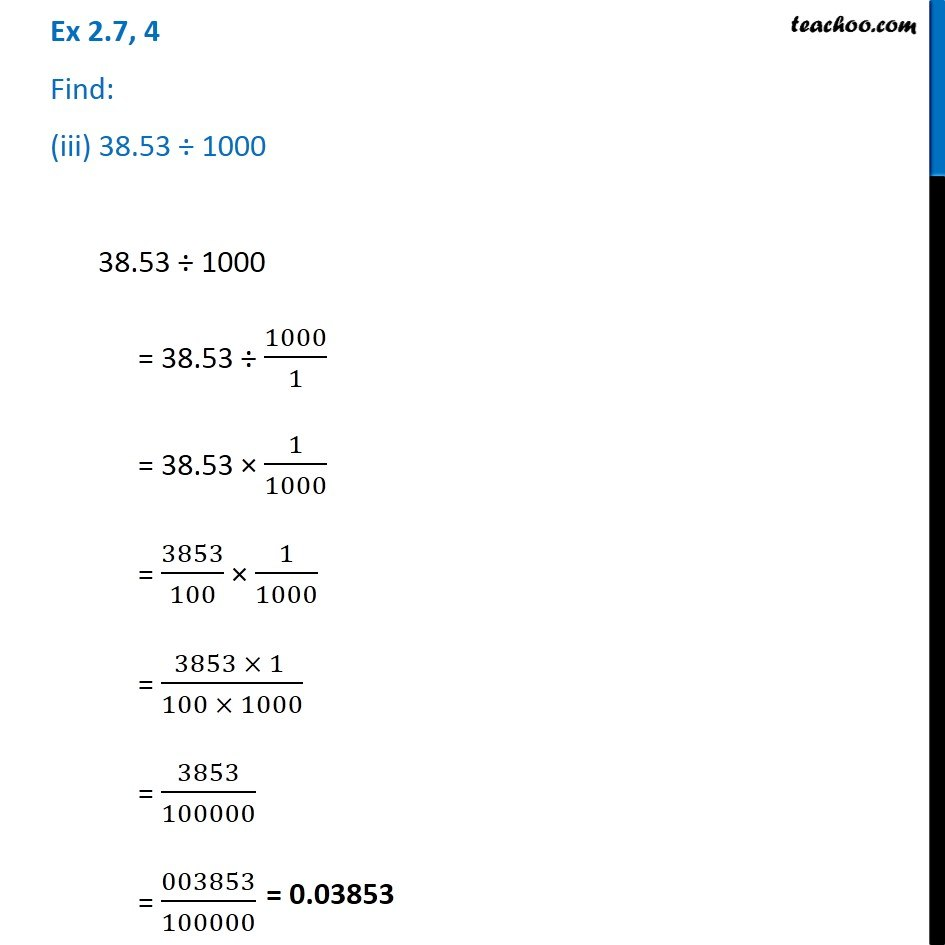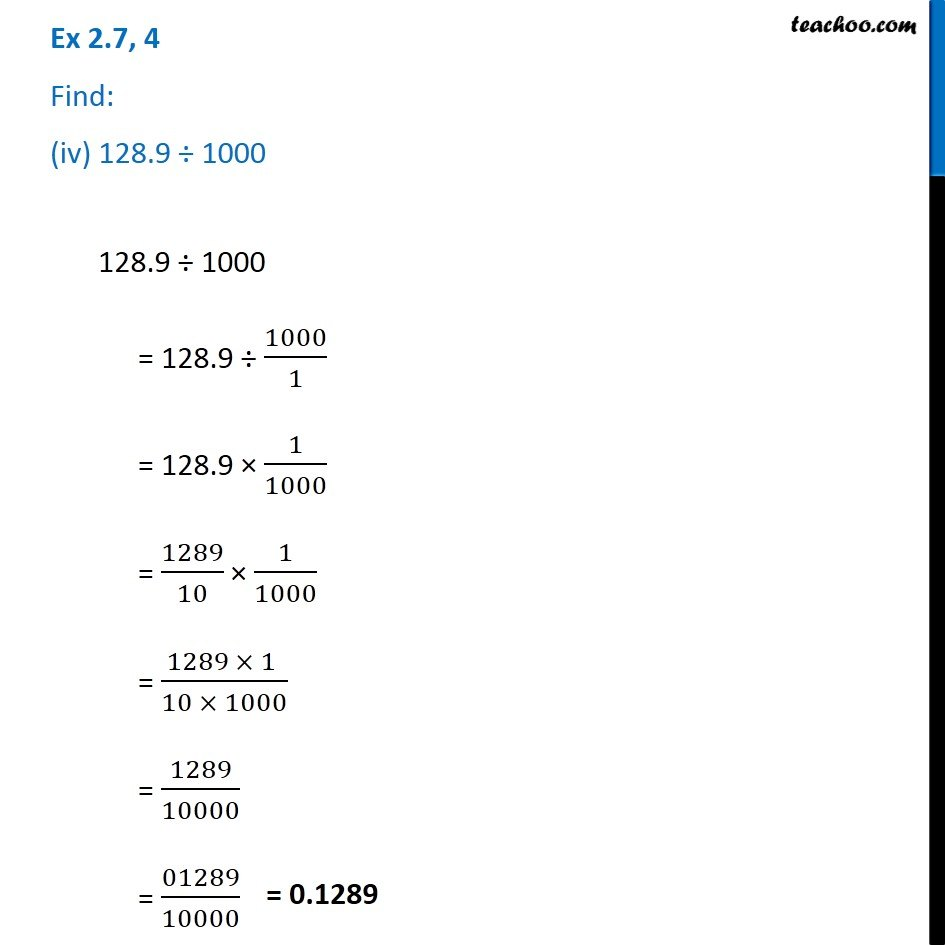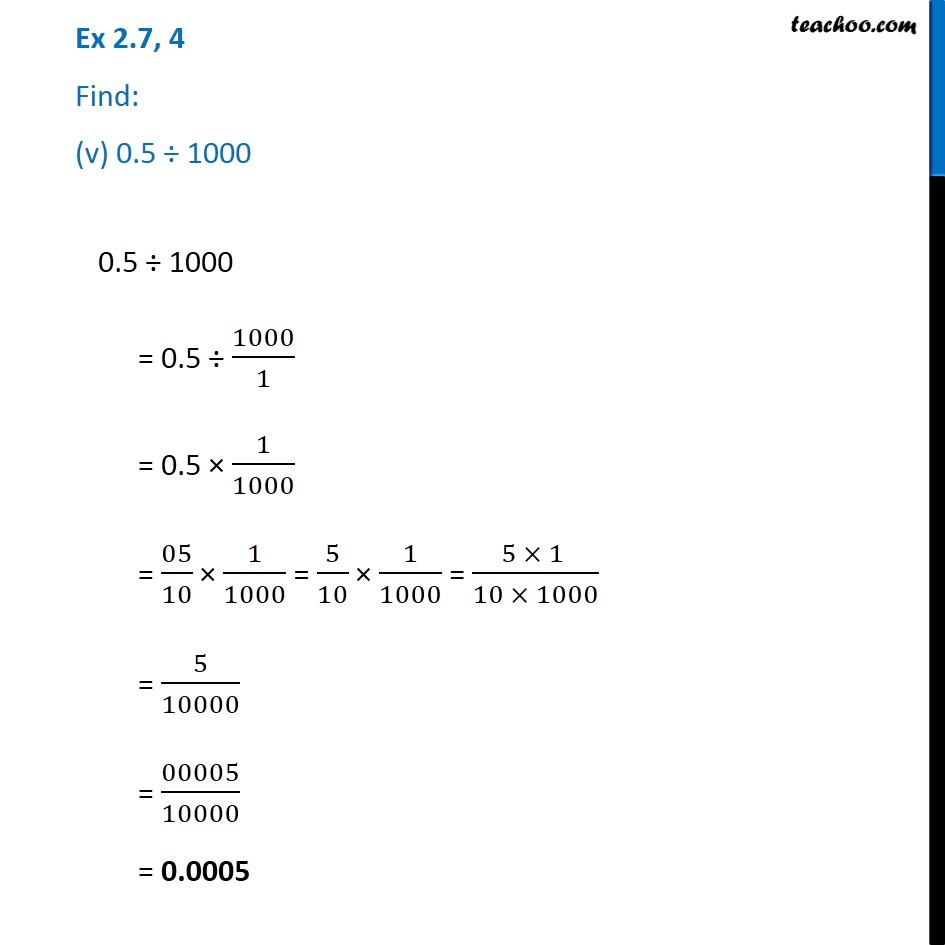Subscribe to our Youtube Channel - https://you.tube/teachoo

1. Chapter 2 Class 7 Fractions and Decimals
2. Concept wise
3. Division of Decimals

Transcript

Ex 2.7, 4 Find: (i) 7.9 ÷ 10007.9 ÷ 1000 = 7.9 ÷ 1000/1 = 7.9 × 1/1000 = 79/10 × 1/1000 = (79 × 1 )/(10 × 100) = 79/10000 = 00079/10000 26.3 ÷ 1000 = 26.3 ÷ 1000/1 = 26.3 × 1/1000 = 263/10 × 1/1000 = (263 × 1 )/(10 × 1000) = 263/10000 = 00263/10000 Ex 2.7, 4 Find: (iii) 38.53 ÷ 100038.53 ÷ 1000 = 38.53 ÷ 1000/1 = 38.53 × 1/1000 = 3853/100 × 1/1000 = (3853 × 1 )/(100 × 1000) = 3853/100000 = 003853/100000 Ex 2.7, 4 Find: (iv) 128.9 ÷ 1000128.9 ÷ 1000 = 128.9 ÷ 1000/1 = 128.9 × 1/1000 = 1289/10 × 1/1000 = (1289 × 1 )/(10 × 1000) = 1289/10000 = 01289/10000 Ex 2.7, 4 Find: (v) 0.5 ÷ 10000.5 ÷ 1000 = 0.5 ÷ 1000/1 = 0.5 × 1/1000 = 05/10 × 1/1000 = 5/10 × 1/1000 = (5 × 1 )/(10 × 1000) = 5/10000 = 00005/10000 = 0.0005

Division of Decimals